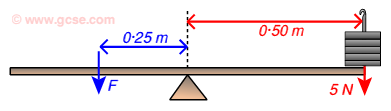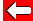# Balancing Moments

One force on its own isn't much use to us. We normally look at situations where turning effects are balanced (or not!).

Let's look at the example below and find the missing force F:If the system is balanced, the anticlockwise turning effect of force F must equal the clockwise turning effect:

clockwise moment = anticlockwise moment

Clockwise moment = 5 N × 0·50 m = 2·50 Nm.

Anticlockwise moment = F × 0·25 m = 2·50 Nm
Force F = 2·50 Nm ÷ 0·25 m = 10 N

In order to balance the 5 N force acting at 0·5 m from the pivot, we require 10 N acting in the opposite direction but at 0·25 m.Go back a pageForces & Motion MenuGo to next page